# Ninja Dollar - LCD game

A simple and fun LCD game.

IntermediateFull instructions provided13,774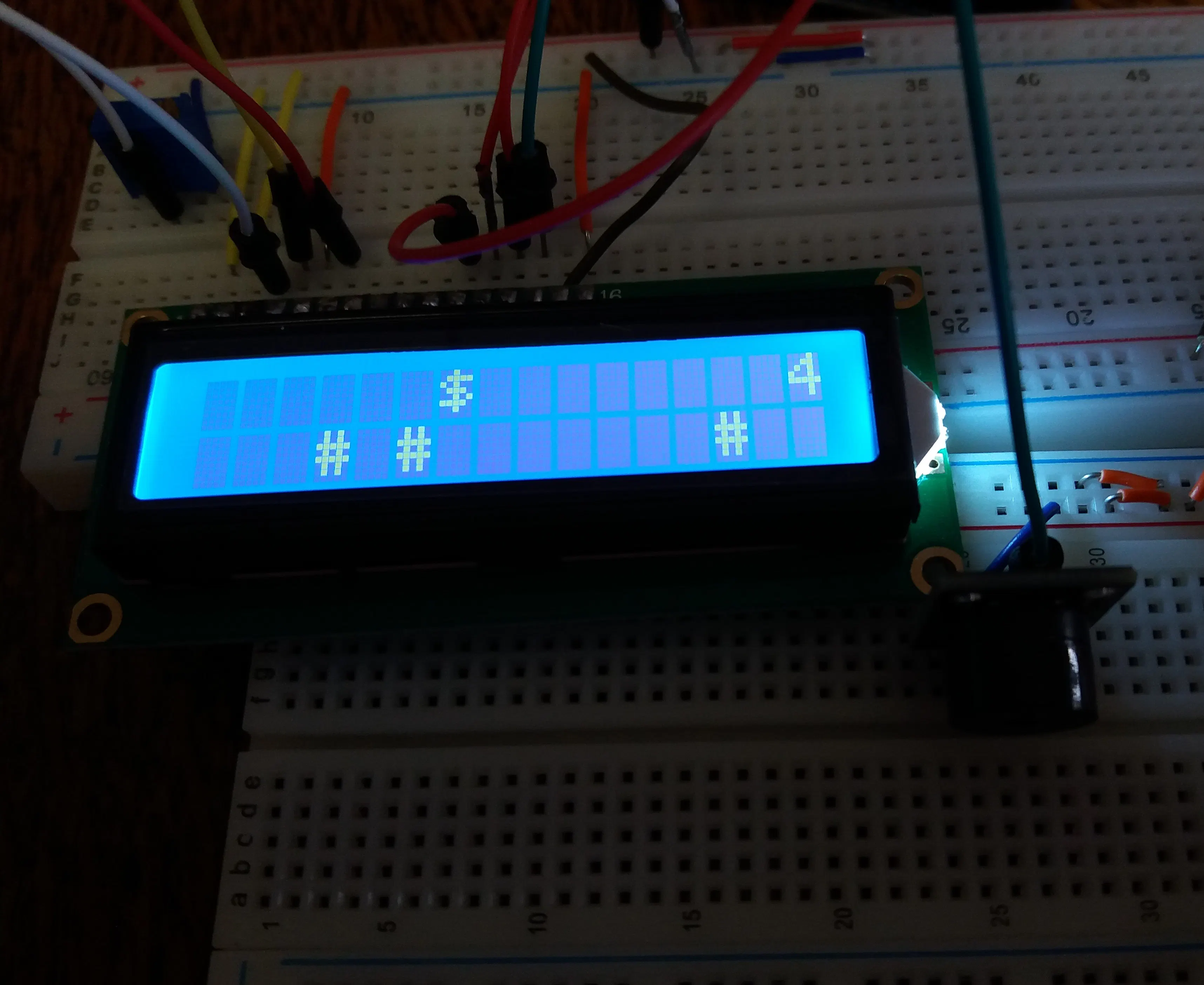## Things used in this project

### Hardware componentsArduino UNO
×1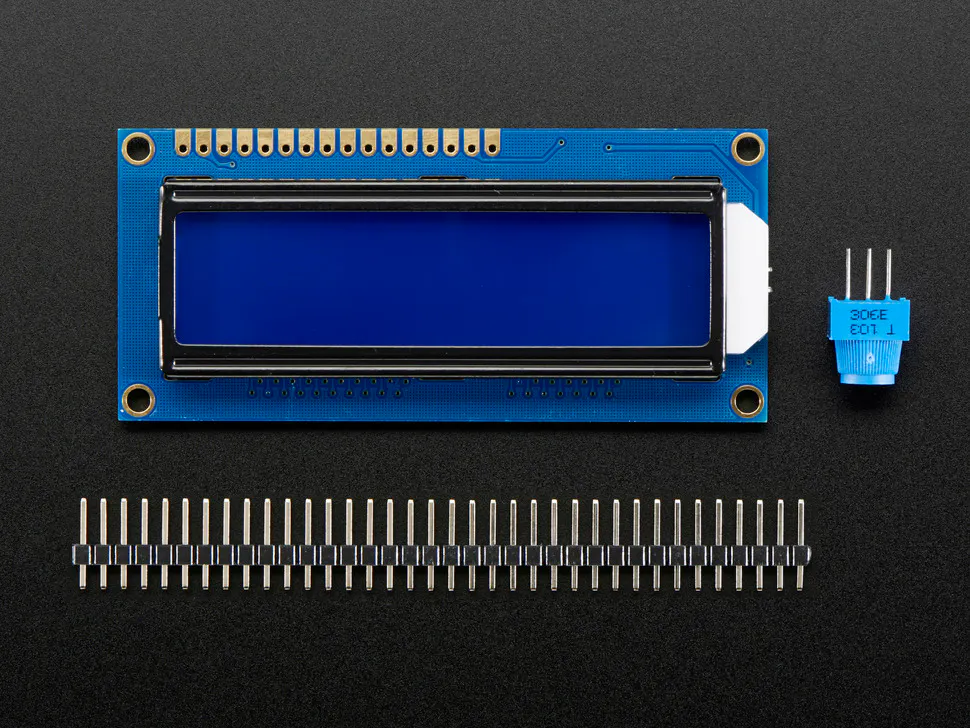Adafruit Standard LCD - 16x2 White on Blue
×1Breadboard (generic) I used two for more space, you can use only one breadboard.
×2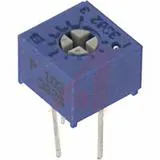Single Turn Potentiometer- 10k ohms
×1LED (generic)
×2Resistor 1k ohm
×4SparkFun Pushbutton switch 12mm
×2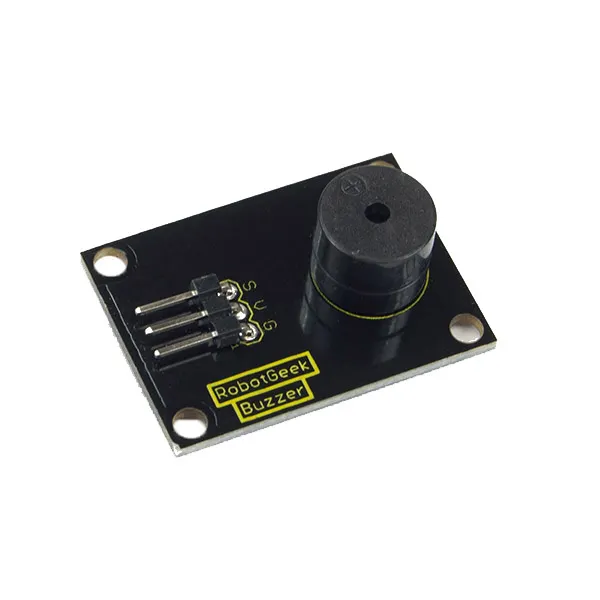RobotGeek Buzzer
×1Jumper wires (generic)
×1

### Hand tools and fabrication machinesSoldering iron (generic) For soldering LCD

## Schematics

### dollar_warrior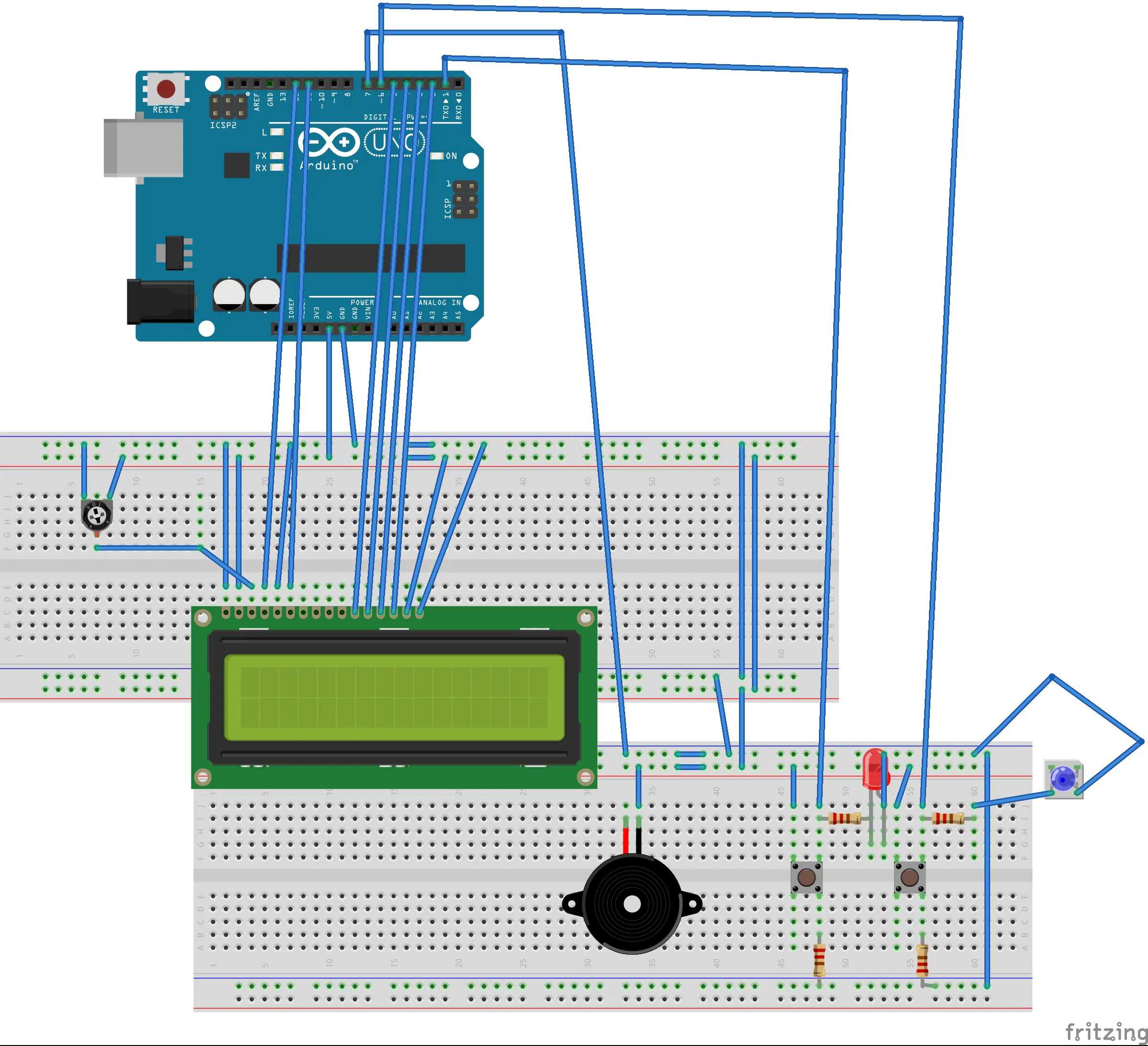## Code

### dollar_warrior

Arduino
```#include <LiquidCrystal.h>

const int rs = 12, en = 11, d4 = 5, d5 = 4, d6 = 3, d7 = 2;
const int buttonPin1=1;
const int buttonPin2=6;
const int buzzer=7;
unsigned long pts=0;
//set buttonstates
bool buttonState1=0;
bool buttonState2=0;
//random number for position of obstacles
int randomNums;
//random number for number of obstacles
int randomNum=0;
//random number for position of pts
int randomNums1;
//random number for number of pts
int randomNum1=0;
//start delay time, which decreases gradually
unsigned int myDelay=500;
//made this boolean to check if button2 is pressed because if it's pressed once in the first for loop i want obstacles not to be written until the end of it
bool temp=0;
//this variable stores the positions of the warrior while he shoots. there can be 16 positions because the warrior has 16 positions.
int tempI;
//i use this boolean to check if the point is catched
bool temp1=0;

//use this variable to store the position of the catched point. it must be and array to store the position of all the points in one iteration of the first for loop. if it stored just one position, then the "old" point would come back in the new iteration
int tempI1;
//use this variable to have a number of shots of the warrior, which is also the length of an array tempI
int button2IsPressed=0;
LiquidCrystal lcd(rs, en, d4, d5, d6, d7);

void setup() {
// set up the LCD's number of columns and rows:
lcd.begin(16, 2);
// set buttonpin mode
pinMode(buttonPin1,INPUT);
pinMode(buttonPin2,INPUT);
pinMode(buzzer,OUTPUT);
lcd.setCursor(4,0);
lcd.print("THE GAME");
lcd.setCursor(2,1);
lcd.print("STARTS IN ");
lcd.print("5");
delay(1000);
lcd.setCursor(12,1);
lcd.print("4");
delay(1000);
lcd.setCursor(12,1);
lcd.print("3");
delay(1000);
lcd.setCursor(12,1);
lcd.print("2");
delay(1000);
lcd.setCursor(12,1);
lcd.print("1");
delay(1000);
lcd.clear();

}

void loop() {
here:

randomNum=random(5);
for(int i=0; i<randomNum; ++i){
randomNums[i]=random(16);}
randomNum1=random(3);
for(int i=0; i<randomNum1; ++i){
randomNums1[i]=random(16);
}
//i reset temp variable before every new for loop because the cycle of the moving warrior starts again
temp=0;
for(int j=0; j<3; ++j){
tempI1[j]=0;
}
button2IsPressed=0;
//loop that writes dollar(warrior) on the lcd which jumps everytime button is pressed
for(int i=0; i<16; ++i){

//pts must be written here because of the lcd.clear() below (pts wouldn't be written down the whole time otherwise)
//i must check if pts is greater than 9 or 99 etc to know how many gaps i should  leave for the number. the more points are collected, the smaller delay time is and the faster is dollar
if(pts>9 && pts<20)
{lcd.setCursor(14,0);
myDelay=400;
}
else if(pts>19 && pts<30) {
lcd.setCursor(14,0);
myDelay=300;
}
else if(pts>29 && pts<50)
{
myDelay=200;
lcd.setCursor(14,0);
}

else if(pts>=50){
pts=0;
myDelay=500;
lcd.clear();
lcd.setCursor(5,0);
lcd.print("VICTORY");
tone(buzzer,262);
delay(200);
tone(buzzer,330);
delay(200);
tone(buzzer,392);
delay(100);
tone(buzzer,330);
delay(100);
tone(buzzer,392);
delay(100);
tone(buzzer,523);
delay(200);
noTone(buzzer);
delay(3000);
lcd.clear();
goto here;
}
else lcd.setCursor(15,0);
lcd.print(pts);

//setting obstacles if the warrior didn't shoot or he shot but he also jumped
if(!temp){
for(int j=0; j<randomNum; ++j){
lcd.setCursor(randomNums[j],1);
lcd.print("#");
}
}
//checking if the warrior  had shot but there were obstacles before him, we want those obstacles to stay
else{
for(int j=0; j<randomNum; ++j){
//i check only the tempI position because that's the when the warrior shot for the first time and he removed all the remaining obstacles
if(tempI>randomNums[j]){
lcd.setCursor(randomNums[j],1);
lcd.print("#");

}

}
}

//setting pts
if(!temp1){
for(int j=0; j<randomNum1; ++j){
lcd.setCursor(randomNums1[j],0);

lcd.print("*");

}
}
else{
for(int j=0; j<randomNum1; ++j){

if(randomNums1[j]!=tempI1[j]){
lcd.setCursor(randomNums1[j],0);
lcd.print("*");
}
}
}

//if the button is pressed we set the cursor up (so that our warrior jumps)
if(buttonState1==HIGH)
{lcd.setCursor(i,0);
tone(buzzer,131);
delay(200);
noTone(buzzer);
}
else lcd.setCursor(i,1);

lcd.print("\$");

//if button2 is pressed our warrior shoots. i had to put this loop here so that warrior shoots from his current spot and then continues to move. that's why delay time is 5 so that this loop finishes as soon as possible
if (buttonState2==HIGH)
{
tone(buzzer,175);
delay(100);
noTone(buzzer);

temp=1;
//if the button1 is low then we remove all the obstacles. that's why the state if temp variable is changed
if(buttonState1==LOW)
{

tempI[button2IsPressed]=i;
}
++button2IsPressed;
for(int k=i+1; k<16; ++k){
//if the buton1 is low then warrior shoots in the second row. otherwise it shoots in the first row
if(buttonState1==LOW)
lcd.setCursor(k,1);
else lcd.setCursor(k,0);
lcd.print("~");
delay(5);
if(buttonState1==LOW)
lcd.setCursor(k-1,1);
else lcd.setCursor(k-1,0);
lcd.print(" ");
delay(5);

}
}

delay(myDelay);
lcd.clear();
//checking if the positions of the pts and the warrior are the same and if the button is pressed because then they collide and we gain 5 extra points
for(int j=0; j<randomNum1; ++j){
if(i==randomNums1[j] && buttonState1==HIGH){
temp1=1;
tempI1[j]=i;
pts+=5;
}
}

//checking if the positions of the obstacle and the warrior are the same and if the button1 is not pressed, then they collide and it is the end of the game
for(int j=0; j<randomNum; ++j){
if(i==randomNums[j] && buttonState1==LOW && temp==0) {

pts=0;
myDelay=500;
lcd.clear();
lcd.setCursor(6,0);
lcd.print("GAME");
lcd.setCursor(6,1);
lcd.print("OVER");
tone(buzzer,349);
delay(200);
tone(buzzer, 277);
delay(200);
tone(buzzer,220);
delay(100);
tone(buzzer,277);
delay(100);
tone(buzzer,220);
delay(200);
noTone(buzzer);
delay(3000);
lcd.clear();
//i must skip the for loop because otherwise the game would continue where it ended
goto here;

}
//counting number of skipped obstacles and that would be our points
else if(i==randomNums[j] && buttonState1==HIGH) ++pts;
}

}

}
```

## Credits

### anime

1 project • 3 followers